# Calculate Mean & Standard Deviation in SPSS

This quick tutorial will teach you how to calculate the mean and standard deviation of a set of data in SPSS. There are a number of different ways to achieve this, but what follows is probably the easiest.

### Quick Steps

1. Click Analyze -> Descriptive Statistics -> Descriptives
2. Drag the variable of interest from the left into the Variables box on the right
3. Click Options, and select Mean and Standard Deviation
4. Press Continue, and then press OK
5. Result will appear in the SPSS output viewer

## The Data

We’re working on the assumption that you have already imported your data into SPSS, and you’re looking at something a bit like this (though obviously with different variables, figures, etc).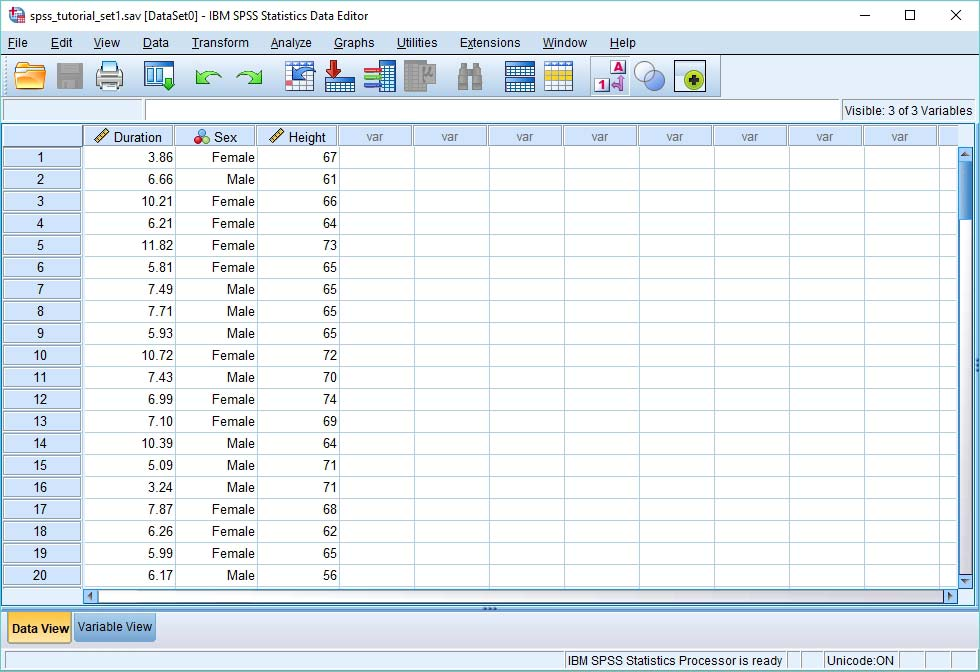As you can see, we’ve got three variables: (a) duration – which is the duration in seconds it takes to complete a certain task; (b) sex – male or female; and (c) height – in inches. You want to find out the mean and standard deviation of the duration variable. In other words, you want to know the average time it took to do the task, and how much the times vary – their spread.

## The Calculation

To calculate the mean and standard deviation, choose Analyze -> Descriptive Statistics -> Descriptives, as below.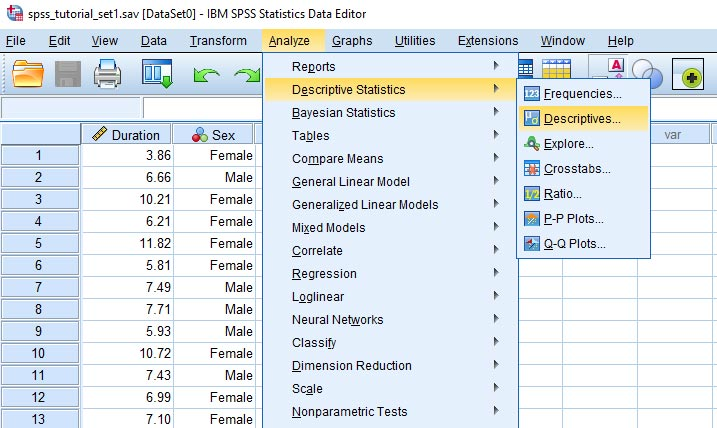This will open up the following dialog box.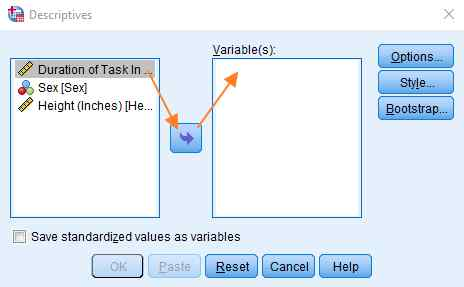You need to get the variable for which you want to know the mean and standard deviation into the variables box on the right (as per the image above). This can be done by selecting it on the left, and then clicking the blue arrow button.

Now choose Options, and select Mean and Std. deviation (and if you wish you can choose additional measures – e.g., Skewness).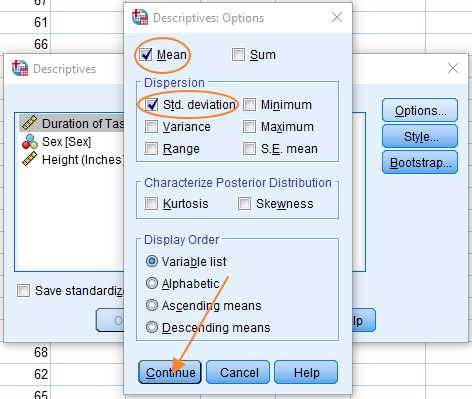That’s pretty much it. Press Continue, and then press OK in the Descriptives dialog box.

## The Result

The SPSS output viewer will appear with the following result (though, of course, the result will be different according to the data you enter).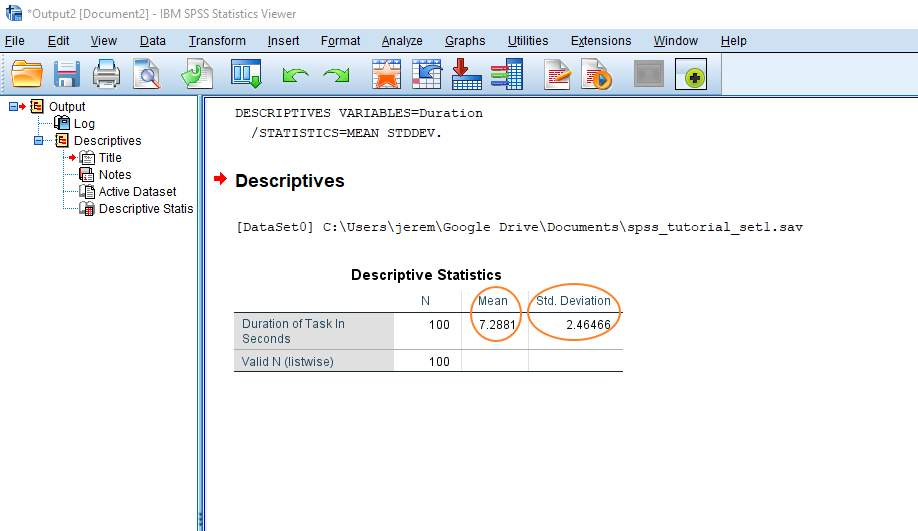As you can see, the values for the mean and standard deviation appear next to the value for N (which is the number of items in your dataset).

***************

That’s it for this quick tutorial. You should now be familiar with one method for calculating the mean and standard deviation within the SPSS statistics package.

***************